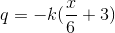# AP Calculus BC : Equations Involving Derivatives

## Example Questions

### Example Question #1 : Equations Involving Derivatives

The temperature profile of a system is given by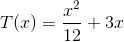The heat flux is given by Fourier's law,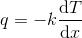, where k is a constant.

Find the heat flux for the given profile.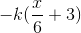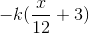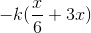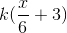Explanation:

To find the heat flux, we must find the change in temperature with respect to x: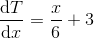The derivative was found using the following rule: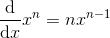Plugging this into Fourier's law, we get